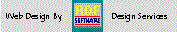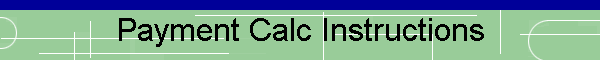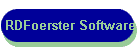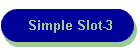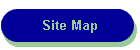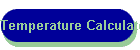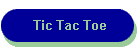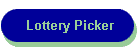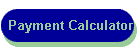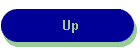RDF-Home

LUGIE
Linux Users Group

How does it work: The payment calculator can calculate a result for any unknown from among the four required parameters; principal, rate, term {years} and payment amount.  To minimize entry requirements and to allow "what if?" usage current parameters are not cleared after each calculation.  Remember to enter a zero {not a space} for any parameter you want to have as the 'unknown".You can also specify the month number of the first payment and give the loan a name to identify it.  These two parameters are helpful if you wish to print out a schedule of payments.It will also handle calculations for repayment schedules that are some period other than monthly.

Testing Results: Once you have calculated for an unknown parameter, you can test it   by recalculating with the new value and set one of the other known parameters to zero to determine if the test calculation matches the known you just replaced with zero.

Printing a Schedule of Payments: When you are satisfied with the parameters of the loan, you can view a schedule of payments on your screen, and if the final version, it can be printed out on your printer or saved as an '.htm' file via your browser.

Usage Scenarios:

1 -  You are told how much your payments, term and the interest rate are.  How much  are you borrowing??   You can use this to check whether the price you expect to pay, less any down payment, agrees with this calculation of the amount you are borrowing.  it should be very close.  Generally, you should round the principal amount result you obtain with this calculator to the nearest dollar.  Follow these steps:

1. Enter: Payment Amount
2. Enter: Interest Rate
3. Enter: Years {term of loan}
4. Enter: 0 {zero} in Principal Amount
5. Check number of periods per year {12=monthly}
6. Press Calculate

2 -  You are told how much your payments, term and the principal amount are.  How much  is your interest rate??  You can use this to determine and compare the interest of the loan.  This calculation is an estimate of the interest rate.   Follow these steps:

1. Enter: Principal Amount
2. Enter: Payment Amount
3. Enter: Years {term of loan}
4. Enter: 0 {zero} in Interest Rate
5. Check number of periods per year {12=monthly}
6. Press Calculate

3 -  You need to borrow an amount, and know the interest rate that will be charged.  How long will it take to repay the loan if the payments are what you would like to pay??   You can also use this to determine and compare how increasing or decreasing the payment amount affects the time it takes to repay the loan.    Follow these steps:

1. Enter: Principal Amount
2. Enter: Payment Amount
3. Enter: Interest Rate
4. Enter: 0 {zero} in Years {term of loan}
5. Check number of periods per year {12=monthly}
6. Press Calculate

4 -  You need to borrow an amount, and know the interest rate that will be charged, and the number of years you can take to repay the loan.  What are my payments.    You can also use this to determine and compare how increasing or decreasing the interest rate affects your repayment amount.   Follow these steps:

1. Enter: Principal Amount
2. Enter: Interest Rate
3. Enter: Years {term of loan}
4. Enter: 0 {zero} in Payment Amount
5. Check number of periods per year {12=monthly}
6. Press Calculate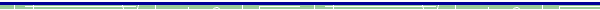dBase, Delphi  and C++ Builder  are trademarks of Borland International, Inc. All other products mentioned are registered trademarks or trademarks of their respective companies. Questions or problems regarding this web site should be directed to RDF@RDFoerster.com. Copyright © 1997-2007 RDFoerster Software. All rights reserved. Last modified: Sunday June 04, 2006